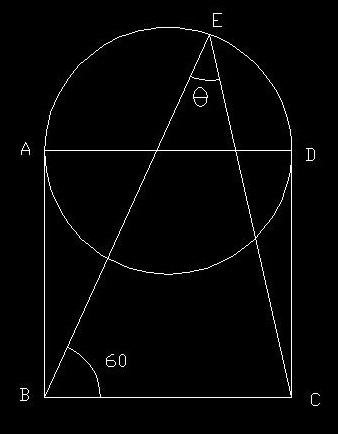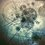# HELP: what is value of the angle?ABCD is a square and AD is the diameter of the circle. what is the value of $\theta$ ?

I think $\theta$ is independent of the length of the sides of the square and am getting an answer approximately 40.183 degrees. I got the answer by solving some properties of triangle equations and doing some constructions. I don't know whether it is right or wrong. My question is what is the exact angle? And can we get the angle by means of constructions only (I mean without using a calculator because in my case I needed to calculate huge surds:-\ )?Note by Ankan Bairagi
2 years, 9 months ago

This discussion board is a place to discuss our Daily Challenges and the math and science related to those challenges. Explanations are more than just a solution — they should explain the steps and thinking strategies that you used to obtain the solution. Comments should further the discussion of math and science.

When posting on Brilliant:

• Use the emojis to react to an explanation, whether you're congratulating a job well done , or just really confused .
• Ask specific questions about the challenge or the steps in somebody's explanation. Well-posed questions can add a lot to the discussion, but posting "I don't understand!" doesn't help anyone.
• Try to contribute something new to the discussion, whether it is an extension, generalization or other idea related to the challenge.

MarkdownAppears as
*italics* or _italics_ italics
**bold** or __bold__ bold
- bulleted- list
• bulleted
• list
1. numbered2. list
1. numbered
2. list
Note: you must add a full line of space before and after lists for them to show up correctly
paragraph 1paragraph 2

paragraph 1

paragraph 2

[example link](https://brilliant.org)example link
> This is a quote
This is a quote
    # I indented these lines
# 4 spaces, and now they show
# up as a code block.

print "hello world"
# I indented these lines
# 4 spaces, and now they show
# up as a code block.

print "hello world"
MathAppears as
Remember to wrap math in $$ ... $$ or $ ... $ to ensure proper formatting.
2 \times 3 $2 \times 3$
2^{34} $2^{34}$
a_{i-1} $a_{i-1}$
\frac{2}{3} $\frac{2}{3}$
\sqrt{2} $\sqrt{2}$
\sum_{i=1}^3 $\sum_{i=1}^3$
\sin \theta $\sin \theta$
\boxed{123} $\boxed{123}$

Sort by:

Suppose the side length of the square is 1. The circle equation is:

$\Big(x - \frac{1}{2} \Big)^2 + \Big(y-1 \Big)^2 = \frac{1}{4}$

Suppose point B is the origin. Define a direction vector:

$v_x = cos \, 60^\circ \\ v_y = sin \, 60^\circ$

Substitute into the circle equation:

$\Big(\alpha \, v_x - \frac{1}{2} \Big)^2 + \Big(\alpha \, v_y-1 \Big)^2 = \frac{1}{4}$

The remaining steps are:
1) Expand to get a quadratic equation in $\alpha$
2) Solve for $\alpha$. Note that you must solve for the greater alpha value
3) $(E_x, E_y) = (\alpha \, v_x, \alpha \, v_y)$
4) Calculate vectors from E to B and E to C
5) Given those two vectors, use the dot product formula to calculate $\theta$. In other words, take the arc-cosine of a quantity equal to the dot product divided by the product of the vector magnitudes.

This yields $\theta = 37.9235^\circ$

- 2 years, 9 months ago

Taking $B$ as the origin is really clever

- 2 years, 9 months ago

Nice solution! I did a mistake in the calculation. The equations were really messy. I am also getting 37.9 degree. Thank you so much!!!!

- 2 years, 9 months ago

I got approximately 37.9235°, but I'm really not sure because the exact formulas get really messy.

First, I put the construction into a coordinate system with the center of the circle at the origin and 1 unit as the radiua of the circle. Every point on this circle can be written as $(\cos \alpha, \sin \alpha)$, where $\alpha$ is the central angle.
Then I considered the slope of line $DE$. It is given as $60^\circ = \sqrt{3}$, but we can also write it as $\frac {\Delta y}{\Delta x} = \frac {\sin \alpha + 2} {\cos \alpha + 1}$. If we set these two equal to each other, we can solve for $\alpha$ and get $\alpha = 2 \arctan\left(- \frac 1 2 + \sqrt{\sqrt{3}-\frac 3 4}\right)$.
Now we can plug this into $E = (\cos \alpha, \sin \alpha)$ and get $E = \left(-\frac34+\frac12\sqrt{\sqrt{3}-\frac34}, -\frac12+\frac14\sqrt{3}+\frac12\sqrt{\sqrt{3}-\frac94}\right)$.
Then we can calculate the slope of line $EC$ as $\frac {6+\sqrt{3}+\sqrt{12\sqrt{3}-9}} {-7+2\sqrt{3}+\sqrt{4\sqrt{3}-3}}$.
If we take the inverse tangent of this we get the angle at $C$ which we subtract from $180^\circ - 60^\circ$ to get our final answer of $\frac{2\pi}3+\arctan\left(-\frac94-\sqrt{3}-\frac12\sqrt{\frac{81}4+12\sqrt{3}}\right) \approx 37.9235^\circ$.

As I said, it gets really complicated and I'm not sure if I've calculated it correctly but I think the way I did it is right.

- 2 years, 9 months ago

I got the same answer. My solution is above

- 2 years, 9 months ago